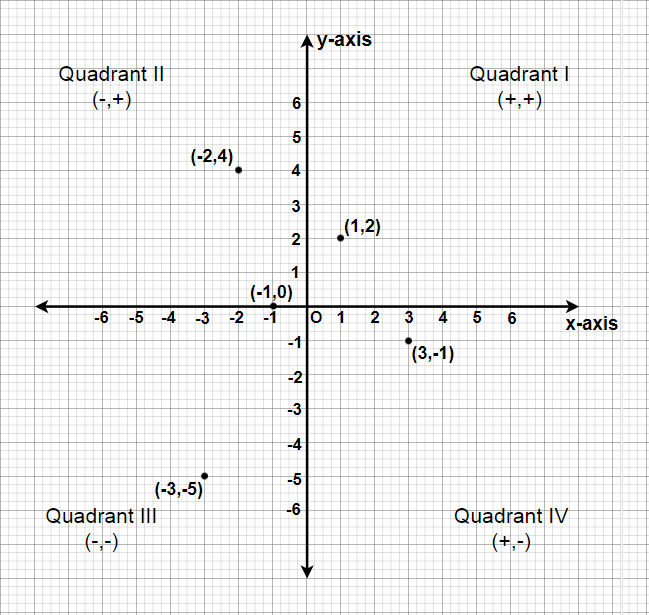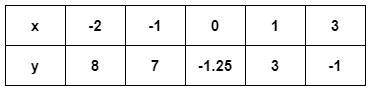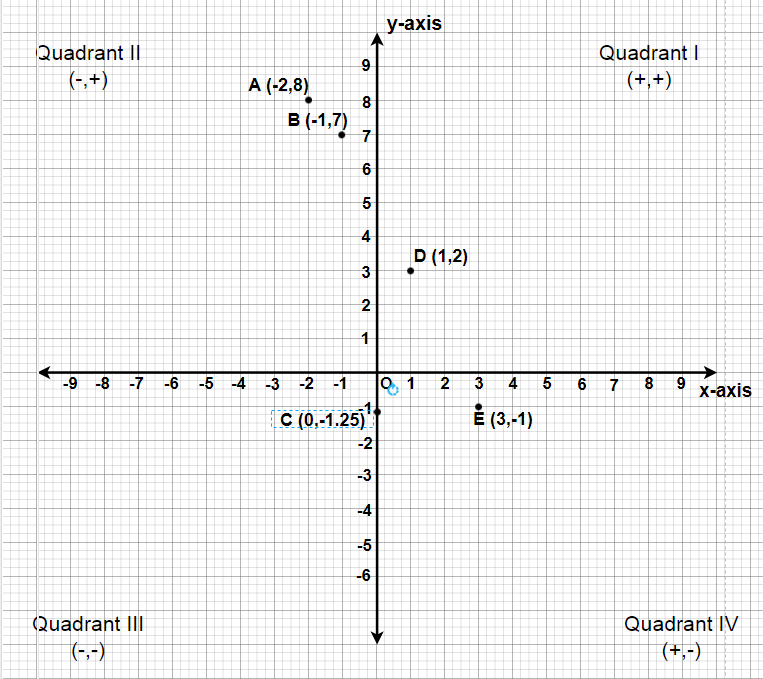Open in App
Not now

# Class 9 NCERT Solutions – Chapter 3 Coordinate Geometry – Exercise 3.3

• Last Updated : 23 Feb, 2021

### Question 1: In which quadrant or on which axis do each of the points (– 2,  4), (3,  – 1), (– 1,  0), (1,  2) and (– 3,  – 5) lie? Verify your answer by locating them on the Cartesian plane.

Solution:(i) The point (-2, 4) lies in IInd Quadrant in the Cartesian plane as x coordinate is negative and the y coordinate is positive.

(ii) The point (3, -1) lies in IVth Quadrant in the Cartesian plane as x coordinate is positive and the y coordinate is negative.

(iii) The point (-1, 0) lies on the negative x-axis and the value of x coordinate is negative.

(iv) The point (1, 2) lies in Ist Quadrant in the Cartesian as both x and y are positive.

(v) The point (-3,-5) lies in the IIIrd Quadrant in the Cartesian plane as both x and y are negative.

### Question 2: Plot the points (x, y) given in the following table on the plane, choosing suitable units of distance on the axes.Solution:

We have to plot these points A(-2, 8), B(-1, 7), C(0, -1.25), D(1, 3) and E(3, -1).
Steps we have to use to plot these points,

• Let 1 unit represents 1 cm.
• To plot (-2, 8), we take (-2) units on x-axis and (+8) units on y-axis. Now we can plot A (-2, 8), it will lie in quadrant-II.
• To plot (-1, 7), we take (-1) units on x-axis and (+7) units on y-axis. Now we can plot B(-1, 7), it will lie in quadrant-II.
• To plot (0, -1.25), we will proceed (-1.25) units under the x-axis on the y-axis and mark the plot as C(0, -1.25), it will lie on the negative side of y-axis.
• To plot (1, 3), we take (+1) unit on x-axis and (+3) units on y-axis. Now we can plot D(1, 3), it will lie in quadrant-I.
• To plot (3, -1), we take (+3) units on x-axis and (-1) unit on y-axis. Now we can plot the point E(3, -1), it will lie in quadrant-IV.My Personal Notes arrow_drop_up